// The Frrraction Guide to Fraction Math  ↑ to Frrr102 Menu  ↑ to Main Menu

### Decimal fractions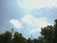Decimal Background: Frrraction was originally about nothing but exact numbers—modular integers and exact fractions. No longer: The inexact numbers called decimal fractions, or just decimals, are so numerically useful that Frrraction now fully embraces decimals alongside the residues and fractions. Complete background on the origin of Frrraction is here.

There are bridges between fractions and decimal fractions. One of them is a really powerful thing Frrraction can do with decimal numbers, but first let's discuss the ordinaries.

### Decimal display of fraction results

Quick version:
The decimal value of fraction results is shown—to eight digits—in the Hden cell (H1den or H2den) beside the denominator part of the active fraction.Connections between decimal fractions and stacked fractions: One bridge between decimals and fractions shows up when you put Frrraction into mixed fraction mode and perform arithmetic or use the GCD function: The decimal number equivalent of the result is displayed in a cell called H1den or H2den, located in the active fraction beside the denominator-part F1den or F2den of the result. In the case of GCD, both H1den and H2den are displayed, no matter which was the active fraction that got reduced. But, calling Hden the decimal number is misleading. To be accurate one should say, "a portion of the equivalent decimal number is displayed in Hden." That's because decimal numbers are infinitely long. For instance, the fraction 12 + 543/999 can be expressed as 12.543, 12.543543, 12.543543543, ... So all we can show is a finitely long leading portion of any decimal number. Likewise, when one says a decimal number is 12.5 the number they are talking about might be any of 12.5000, 12.54, 12.47300, and so on. The notation '12.5' tells you nothing about the omitted digits.

### More than meets the eye

Quick version:
Decimals have an infinite number of digits.
Sometimes most of them are 0. Sometimes not.How many decimal digits?: If we're given a long decimal then all the shorter ones are definitely known. We just choose how many "significant digits" we wish. Those three decimal expressions for 12 + 543/999 in the previous Background had respectively 5, 8, and 11 digits. Given a decimal with many digits, we can specify a smaller number of digits and use one of two ways to perform the shortening: round or truncate. For example, say we have the 11-digit decimal version 12.543543543 of 12 + 181/333 and we want a 5-digit version. We can truncate at 5-digits by simply discarding all the portion to the right of the fifth, yielding 12.543. The other way is to round it off: Look at the fifth and the sixth digits; if the sixth is 0,1,2,3,or 4, leave the fifth unchanged, just truncate; but if the sixth is 5,6,7,8, or 9 then add 1 to the fifth, round up. In the present example, the 5-digit rounded version is 12.544 and the 6-digit version is 12.5435. We worked the example by examining and editing the displayed text of the decimal number. That's easy for humans, but more time-consuming to simulate on a computer. Here is a purely numeric process for doing the same job.

### ⋅ (the Dot key) puts decimal point —and more

Key idea

While entering a number into and Fcell, tapping ⋅ (the Dot key) will enter a decimal point at the cursor location. The containing Fcell and its mate in the other fraction then work as a decimal pair for arithmetic operations.

A bit of history: Before Frrraction's keystrip had a '' (Dot) key, entering dots used to be accomplished by a fast doubletap of the 0-key, which was described in our documentation as "00". Traces of that designation remain, e.g. in the hp( commands 00c and 00put where "00" is intended to remind that the commands are decimal operations.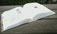More Dot-key decimal actions If you tap the ⋅ Dot key in an Fcell that already contains a decimal point, it changes the exponent of a power-of-ten multiplier. For instance, 32.45 would become 32.45e+0, meaning 32.45 times ten to the power 0. Of course, 100 equals 1 so, numerically, 32.45e+0 = 32.45. Tapping digit keys then modifies the magnitude of the exponent. Tapping the bDel key removes exponent digits or the exponent itself. Tapping ⋅ Dot in an Fcell which already contains an exponent simply changes the +- sign of the exponent, e.g. 32.45e-2 (meaning 0.3245) becomes 32.45e+2 (meaning 3,245.). A technical detail: Arithmetic performed in Frrraction when the user manually touches command keys on the keystrip are called "CF operations"; arithmetic performed within the hp( portions of applets are called "hp( operations". Frrraction's CFdecimal fractions are handled internally in "single precision floating point" format and can handle at most 8 digits. The first 7 digits are quite reliable. The 8th can be off by 2 or more, i.e. 12345.676 might appear as any value between 12345.674 and 12345.678 or worse. Single precision numbers fit comfortably into the fCells utilized by CF operations. hp(decimals handle 38 significant decimal digits, much higher precision than CFdecimals. Display of such higher precision results is best done in the hShow quadrant of Frrraction's MAIN view, controlled by hp instructions within Frrraction applets.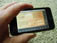Try it: To enter 215.543e-2 you do: clr, tap 2, tap 1, tap 5, tap ⋅ , tap 5, tap 4, tap 3, tap ⋅ , tap 2, then tap ⋅ again. Note that the Fcell's font changed color when you tapped ⋅ the first time (unless the cell's mate already had a decimal point so the cell and its mate in the other fraction were already blue). This can be done in any or all Fcells, with the same effect—creating up to three drcimal pairs.Decimal arithmetic in Frrraction The + - x / arithmetic keys work on pairs of decimals much as they work on pairs of fractions: The active number gets its inactive mate added to it, or subtracted from it, or multiplied by it, or divided into it. The inactive mate remains unaltered. The UNDO gesture recovers the original value of the active number if you don't like the result of the arithmetic. How does Frrraction know when + - x / should be decimal arithmetic on a single pair of Fcells, or should be fraction arithmetic on the complete pair of fractions? Also, when should UNDO RCL and STO manage storage of decimal (one i.f number) or fraction (three integers num, den, and int)? It's a simple answer: + - x / STO RCL and UNDO should all match the nature of the active Fcell. Suppose F2 = 3 + 5/12.3 and the active Fcell happens to be F2den containing the decimal 12.3; then + (add) would decimal-add the contents of F1den to F2den, leaving all Fcells in both F1 and F2 unchanged except F2den, which would hold the new decimal sum. But... In the same starting setup of F2 except the active Fcell is F2int containing the integer 3; then + would fraction-add F1 to F2—ignoring all but the integer part (the 'i' of 'i.f') of each Fcell in the two fractions—leaving F1 unchanged and all cells of F2 newly containing the ?ndi? Fraction-result.Moving decimals within Frrraction: Using the digit keys and bDel, you can edit the decimal fraction in any Fcell. Tapping bDel a few times (or the Clr button once) will remove the decimal point and revert the active Fcell to integer. You can STO and RCL a decimal from or to the active Fcell. Frrraction maintains separate independent storage registers for ndiFractions and decimals. Thus, if you STO or RCL when the active Fcell is a decimal then only that Fcell gets stored or gets changed into the recalled value. On the other hand, if you STO or RCL when the active Fcell is an integer then the whole ndiFraction gets stored or gets changed into the recalled value. XCH and DUP similarly depend upon whether the active Fcell is decimal or integer: If the active Fcell is decimal then the action only involves the specific active Fcell and its mate in the other fraction. If the active Fcell is integer, the action involves all three parts of the fraction: numerator, denominator, and integer-part.

### D|F converts between decimal and ndi:stack formats

Key idea

With the cursor in any Fcell, D|F converts back and forth between active Decimal(inline) fractions and NumDenInt(stacked) fractions.The D|F (Decimal or NumDenIntFraction) yellow function The Y D|F gesture converts fractions between Decimal and NumDenInt Fraction formats. If the active Fcell is an integer, the result is a decimal fraction in the active fCell. On the other hand, if the active fCell is a decimal, then the effect is to replace the whole active fraction by a stacked form of the original flat decimal. One context where this conversion is often useful is during complex calculations. Fraction numerators and denominators tend to grow large during a sequence of arithmetic operations. If you can stand the loss of perfect precision, you can use D|F to flatten the offending stack, then use APRX to find a better stacked fraction to work with. The decimal form of the new fraction of course agrees with the decimal form of the original up to 7-digit accuracy and that's often good enough. The "While You Wait" page on this site is a good example.

### APRX - An exquisite decimal-to-NumDenInt converterFrrraction Procedure

The really powerful thing you can do with a decimal number is to get rid of it: Use the yellow APRX function. APRX is short for 'Approximate', and what it does is generate in the active fraction an efficient stacked version of the decimal number given in the active Fcell—assuming, of course, that the number in the active Fcell actually is part of a decimal pair. (By "efficient" we mean a fraction with small numerator and denominator whose decimal accurately matches the given decimal.)

Decimal-to-Fraction conversion is a surprisingly subtle task. For example, the standard conversion of the famous number e, accurate to 8 digits, is 2 + 7,182,818/10,000,000. To 4 digits it is easily approximated by 2 + 718/1,000. But 2 + 719/1,001 which looks almost the same, is way more accurate (7 digits—off by only 1 in the 8th digit).

You don't figure out stuff like that by a quick calculation in your head!APRX with a decimal in the active fCell

Internally, APRX generates five different efficient decimal-to-stack conversions then selects the best of the five and presents it to you in the active fraction. This can sometimes be very handy.  To get Fig. APRX1, we made F1int be the decimal fraction 12.543 and y-tapped APRX.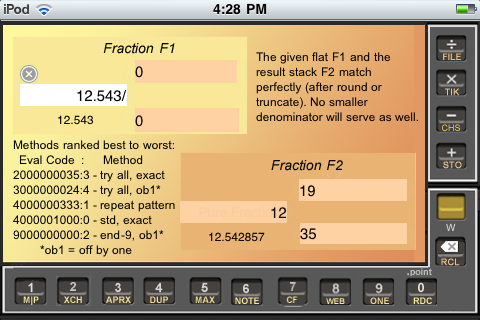Figure APRX1
Summary of all five methods is shown.

The five APRX methods are numbered from 0 to 4 and named "0—std exact", "1—repeat pattern", "2—end-9, ob1", "3—try all, exact", and "4—try all, ob1" where "ob1" is short for "off by 1". For each given target fraction APRX temporarily covers frrrNote to list the methods in order from best to worst performance on that specific fraction, using a numeric "Eval Code". The most significant digit of the eval code shows how many digits are in the denominator (2 for the try-all-exact method, and 4 for the standard method). That digit-count is followed by the actual denominator (000000035 or 35 for Method 3, and 000001000 or 1000 for Method 0).

As you can see, the result (given in Fraction F2) was excellent. The standard mixed form — listed fourth in the ranking — is 12+543/1000, whose denominator is much larger than that of the 12+19/35 that Frrraction's APRX Method 3 found.
Five more APRX Examples
F1intDF2int+F2num/F2den=F2dec
12.512+
1
/
2
=12.5
12.5412+
7
/
13
=12.538462
12.54312+
19
/
35
=12.542857
12.543212+
44
/
81
=12.54321
12.5432112+
44
/
81
=12.54321
In each of the five examples, notice how F2dec correctly rounds off to exactly match all specified digits of F1intD. The standard conversion used by most fraction apps seldom comes close to the efficiency of APRX. The worst case in this series would be 12.54321 = 12 + 54,321/100,000 — six denominator digits for standard vs. two for APRX.

This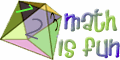links to a page that explains the standard conversion, APRX's "Method 0 std exact". Calling it "9 Recurring", Math is Fun also includes a Special Note explaining part of the APRX "Method 1 repeat pattern".

### CF - Classic Fractionsbackground

CF is short for either "Classic Fraction" or — by far the more generally useful of the two — "Complex Function" a.k.a. "CFunction" — terms meant to distinguish them from Frrraction's built-in yellowkey Functions (+ - MAX EDIT etc.). CFs provide a way to effectively expand the collection of useable keys beyond the sixteen allowed by the limited space on the iPhone screen. The yellow PROG function key gives access to that expansion.

To use CFs, you Edit a FrrrNote to include one or more "CF Brackets" — matched pairs of braces enclosing code that defines the functions.

Within an hNote, the brackets look like this:

CFBracketentries
Egyptian Fraction
{ a0; a1, a2, ... an }
integer denominators
Continued Fraction
[ a0; a1, a2, ... an ]
integer quotients
CFunction
{{ blah this that etc }}
action keywords

Egyptian Fractions and Continued Fractions are similar to each other, and simpler to use than CFunctions, so we discuss them here in HighSchool. Computer Programming is the true subject of Composite Functions so we discuss them in GraduateSchool.

There are two kinds of classic fractions: Continued Fractions and Egyptian Fractions. Continued Fractions are cool for a number of uses, including the secret codes my Grandson Galen and I are doing. Egyptian Fractions built the pyramids 4000 years ago. They're still good for figuring the best way to divvy things up, but aren't useful for much else any more.

An example Continued Fraction is:
5 + 1 / (4 + 1/ (3 + 1/ 2 )).
In normal notation, this continued fraction would be written something like this:

```     1
5 + ——————————————
1
4 + —————————
1
3 + ————
2
```
Continued Fraction Example 1

"Doing the arithmetic" (working up from the lower right) reduces it to the ordinary stacked fraction: 157/30.

Here's an example Egyptian Fraction:
5 + 1/4 + 1/3 + 1/2.
Doing its arithmetic reduces it to the ordinary stacked fraction: 73/12.

Classic Fractions are awkward to type, so Frrraction abbreviates them with a widely used shorthand bracket notation: Square brackets formed with the square braces [ and ] enclose Continued Fractions, and curly brackets formed with the curly braces { and } enclose Egyptian Fractions — look closely, those Egyptian curly braces are not the normal smooth parentheses.

The integer part of the fraction immediately follows the opening brace, and is followed by a semicolon.

If there is no integer part then the first entry within the bracket is 0 followed by a semicolon, viz. [0;a,b].

If the fraction has the mixed form a+0/1 i.e. there is nothing but an integer part then the bracket is simply [a;]. Don't omit the semicolon, though—without it, CF doesn't even recognize <[a] as a continued fraction command.

After the semicolon, the quotients (fraction parts) follow in order, each followed by a comma — although the final comma before the closing brace is usually omitted. For example, the continued fractions [5;4,3,2] and [5;4,3,2,] have integer part 5 and fraction parts 4, 3, and 2 and they equal 157/30.

YTapping Frrraction's EXEC key performs any of several tasks, depending upon what brackets you've put into frrrNote.

• If the current hNote contains an empty square bracket [] then EXEC causes the currently active Fraction to be converted to a Continued Fraction inside that bracket. (If there are several empty brackets, EXEC processes only the one that appears first in the hNote.)
• If, instead, frrrNote contains an empty curly bracket {} then EXEC converts the active Fraction into an Egyptian Fraction inside that bracket. (If there's a mixture of curly and square empty brackets, EXEC loads whichever appears first in the hNote.)
• If there's a loaded CF bracket prefixed with an Enable Code (OK, 'enable code' sounds fancy but it's just the <less-than symbol, or left angle-bracket) , such as <[5;4,3,2] or <{5;4,3,2} then the EXEC function "does the arithmetic" and puts the ordinary fraction into Frrraction's F1 or F2, whichever is currently the active fraction.
• If frrrNote contains no empty brackets and only normal loaded brackets without an enabler code—such as [5;4,3,2] or {5;4,3,2}—or contains no brackets at all, then the EXEC function has nothing to do and issues a complaint.

You don't really need the EXEC function in order to work with classic fractions, of course. You can use normal Frrraction arithmetic or just do the work by hand. Of course, you could wear itchy old hair shirts instead of soft cotton, too.

For example, to convert [5;4,3,2] into an ordinary fraction, the following steps (working from back to front) would do it:

1. put 2 into F1
2. put ONE into F2 then Divide
3. put 3 into F2int (requires Frrraction showing Mixed Fractions)
4. put ONE into F1 then Divide
5. put 4 into F1int
6. put ONE into F2 then Divide
7. put 5 into F2int.

and there you have it: [5;4,3,2] is 5+7/30, or 157/30. (Frrraction's M|P function will convert the mixed fraction 5+7/30 into the pure fraction 157/30 if you don't want to do that chore by hand.)

Here's a procedure to go the other way — convert 157/30 into a continued fraction (writing the bracket down by hand as you go, I suppose):

1. put 0+157/30 into F1
2. Use M|P to convert it to 5+7/30
Now you know that the bracket begins with [5; ].
1. Clear the 5 in F1int to 0
2. put ONE into F2 and Divide.
This leaves 4+2/7 in F2.
1. The bracket continues as [5;4, ].
2. Clear the 4 in F2int to 0
3. put ONE into F1 and Divide.
This puts 3+1/2 into F1.
1. Put the 3 into the bracket as [5;4,3, ]
2. Clear the 3 in F1int to 0
3. put ONE into F2 and Divide.
This puts 2+0/1 into F2.
1. Put the 2 into the bracket as [5;4,3,2, ]
2. Clear the 2 from F2int
This leaves the result as 0+0/1 or just zero, which says we're done. Delete the final comma in the bracket and the answer is complete: 157/30 = [5;4,3,2].

### CF — Secret Code Ring

This is some really fun stuff! (in my humble opinion). If you can find a way to interpret the numbers in a Continued Fraction as letters of the alphabet, then the Continued Fraction becomes a message. And if you convert that Continued Fraction into an ordinary fraction, then the message is quite nicely disguised--especially to people who think numbers are just numbers!

I have been working with one way to interpret numbers as letters: The simple alphaNum code: Replace A by 1, B by 2, ... , and Z by 26. For better communication we extended the simple alphaNum code to include a number for a blank space, a period, and a question mark. We use 32 for space, 46 for . and 63 for ?.

That's all there is to it. Now [0;23,15,23,46] or 1/(23 + 1/(15 + 1/(23 + 1/46))) or 15931/367472 all contain the coded message "WOW." Cool, eh?

After you catch on, you can begin to let Frrraction do the heavy numeric lifting for you: Put the CF command <[0;23,15,23,46] into frrrNote (not forgetting the command arrow '<') and EXEC will put 15931/367472 into the active fraction. Notice that EXEC removes the Command Arrow to let you know it finished the job. Or go the other way: Put 15931/367472 into the active fraction and an empty square bracket [] into frrrNote and EXEC will fill the empty bracket for you, creating the loaded bracket [0;23,15,23,46].

There are two things to avoid:

• Don't end alphaNum-coded messages with the letter A, because A translates to 1 and by tradition Continued Fractions don't end with a one. That's because it would end in a thing like 23 + 1/1, which gets converted to 24 instead. In bracket form, a final 23,1] would get converted to 24]. The end of the message would change from WA into X. Not good.

It goes the other way just as well: If a continued fraction ended in anything but 1, say n + 1/15, this could be written as n + 1/(14 + 1/1) so its bracket then would end with n,14,1]. (This duplicity of terminal 1's is, by the way, the only way that the continued form of a fraction fails to be unique.)
• Don't try to make a long message into a single Continued Fraction. That's because when you convert a long Continued Fraction into a stacked fraction, the numerator and/or denominator of the stacked form can be HUGE, easily bigger than the iPhone's largest number 2147483647. This would cause Overflows in Frrraction when you use the EXEC function to convert between the stack and the continued form.

The solution of this problem is to break long messages into a series of short messages. These short messages are called "codewords" and a good length is four letters per codeword. Don't bother to try to match codeword boundaries to the actual word boundaries in your messages--let the punctuation characters sp . and ? do that job for you.

A typical long message: 16057/16558 28/451 69865/75672 2932/41465 362/1829 1663/1894. The first way I divided it into codewords, two of the codewords ended with A. In each case I just moved the A to the beginning of the next codeword. Those two five-letter codewords didn't cause overflow to occur so there was no other problem.

When you get tired of counting letters of the alphabet to get from messages to numbers, here is Frrraction to the rescue.

The CF Command that converts simpleAlpha to a normal Continued Fraction is: <[@ followed by the alphabetic letters of a codeword and the closing square bracket ]. Use PROG to put that into frrrNote, then employ the EXEC function. Bingo. frrrNote then contains the bracket form of the continued fraction.

For example, if you put <[@SHOW] into frrrNote, then the EXEC function will replace it by [@SHOW] <[0;19,8,15,23,]. So frrrNote would then contain a new CF Command, ready for EXEC to put the numeric continued fraction, which encodes the alphaNum SHOW message, into the active Fraction.

Frrraction can also go the other way: it can decode a stacked fraction that happens to be the alphaNum-encoded form of a text message: The CF Command in frrrNote is just [@] and Frrraction's EXEC function fills the empty bracket with the decoded form of the active fraction.

For example, if the active fraction contains 2791/53375 and [@] is in frrrNote then EXEC will replace [@] by [@SHOW]. If the active fraction was just a number, not an alphaNum-encoded message, what you would most likely get from EXEC is [@~~~~] because the tilde character ~ is what EXEC uses when it doesn't recognize a number as an alphaNum-encoded character.

The next step in Frrraction's Secret Message Codec facility is to use the much more powerful ASCII code instead of the overly simple alphaNum code. The only difference in operation is that the alphaASCII flag is the tilde character ~ rather than alphaNum's @ character. Thus, <[~Wow 012!] converts to [0;87,111,119,32,48,49,50,33,]. The stacked form of that particular numeric continued fraction happens to be so huge it generates an Overflow in Frrraction. The three-to-five character codeword limit applies to ASCII as well as to alphaNum. Breaking it up into two four-letter codewords we get [0;87,111,119,32] = 422831/36790106 and [0;48,49,50,33] = 80932/3886387.

ASCII is Page 1 of UTF8 and Unicode, the world's most important standard communication codes, so well worth being a little familiar with. For reference, here is a convenient list of ASCII codes. Good news is: You do not need to actually know ASCII in order to use it in Frrraction.

Decoding an ASCII-encoded fraction is just as easy: Put an empty ASCII-flagged bracket [~] into frrrNote and the active fraction will be decoded into the empty bracket. For example, if Fraction F2 were active and contained 80932/3886387, the empty bracket would be replaced by F2 = [0;48,49,50,33][~012!] — the numeric continued fraction followed by its ASCII-decoded form.

A final note: The two alpha encodings are mostly incompatible with each other — they share only the space, period, and question mark. I assume that, once you get to the ASCII [~ forms, you won't feel a need to turn back to the simple alphaNum [@ forms.

### CF Example: Best Approximationsbackground

Sometimes you have a fraction whose numbers are too large for the purpose at hand. For instance, maybe you think 177,215/15,979 is a good approximation of √123 but can't test it by simple multiplication in Frrraction because 1772152 overflows. Frrraction's APRX function provides an easy-to-use solution that you already know about — but classic continued fractions provide an unexpected, clever, do-it-yourself alternative.

Example: Suppose you want a simpler fraction (i.e. a fraction whose numerator and denominator are smaller integers) than 1,010/7,009 but with the same 7-digit decimal value. Putting 1,010/7,009 into Fraction F1 and ytapping D|F (Decimal-or-Fraction) puts the decimal form 0.1441004 into whichever is the active fCell in F1. APRX then finds the simpler fraction 712/4,941 which has the decimal form 0.14410038, which rounds to exactly match the seven digits of F1int. That might be a disappointing result, since 712 and 4941 aren't very much smaller than 1010 and 7009, but at least we know it's the best possible—if we want a simpler fraction we'll need to accept fewer than seven digits of precision.

Anyway, the whole point of this CF Example section of the guide is to show that CF continued fractions provide an alternative way to find the most efficient fraction that represents any number in the range from 0.14410035 to 0.144100449, i.e. any number that rounds up or truncates down to the given seven digits. Here's how:Try it. Really. Step 1: Put 0.14410035 —the smallest number that rounds up to match 0.1441004—into F1n and put an empty Continued Fraction bracket [] into frrrNote, then EXEC. This replaces the empty CF bracket by F1 = [0;6,1,15,1,1,3,1,3,8,1,...]. Step 2: Put 0.144100449 —the largest number that rounds down or truncates to match 0.1441004—into F1intD, put another empty bracket [] into frrrNote, and apply CF again. This replaces the empty bracket by [0;6,1,15,1,1,3,1,5,1,8,...]. Step 3: Make a new bracket by copying the previous two as far as they agree, producing [0;6,1,15,1,1,3,1,]. At the next entry, the two brackets disagree: the smaller has a 3, the larger has a 5. Complete your copy by using 1 plus the smaller, viz. 4, so it reads [0;6,1,15,1,1,3,1,4]. Convert that bracket into a command bracket by putting a

Fig. APRX2 summarizes the example. The frrrNote has been edited to include all three steps in one screen. Using frrraction's PROG function, I simply typed the lines "Best Approximation Example" and "1010/7009 = 0.1441004" after doubleclick-00 gave me the decimal value. The lines "F1 = ... = 0.144..." were produced from empty brackets by the CF-command in Step 1 and Step 2. The line "Lesser+1..." shows what's left of the CF-command-bracket <[0;6,1,15,1,1,3,1,4] after Step 3 collapsed it into F2.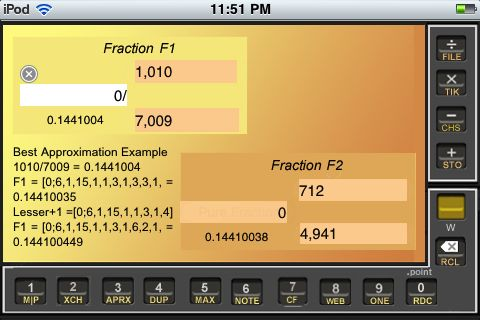Figure APRX2
A CF best-approximation example.Try it. Really.
For a second example, suppose you would be satisfied with a 4-digit approximation 0.1441 for that same fraction used above. Then the above steps become:
 Step 1: 0.14405 → [0;0;6,1,16,...] Step 2: 0.1441499 → [0;0;6,1,14,...] Step 3: <[0;6,1,15] Step 4: → 16/111.
As a check on the result of Step 4, put 0.1441 into F1int; with F1int still active, APRX then yields 16/111 in F2. It always works.Try more: In the above examples we chose intervals to contain precisely all decimals that match (after rounding) a given decimal to a given number of digits. The examples directly generalize to finding the most efficient fraction for a number required only to lie within a specified interval—any interval you want. Here's a little series of problems asking for simplest n/d's in intervals. In each case find the unique fraction n/d, with smallest d which produces a decimal number in the specified range: (a) between 0.123 and 0.456 [I guessed this one to be 1/4=0.25. I was wrong. What's the correct answer? Can you find an interval like these for which the correct answer would truly be 1/4?] (b) between 0.123 and 0.246 (c) between 0.123 and 0.199 (d) between 0.123 and 0.165 (e) between 0.123 and 0.142857 (f) between 0.123 and 0.124999 (g) between 0.123 and 0.12306 The answers in reversed (g)-thru-(a) order are: 31/252, 8/65, 1/8, 1/7, 1/6, 1/5, 1/3.

This starter reference on best approximations explains in more detail.